Courses

# Power Series Mathematics Notes | EduRev

## Mathematics: Power Series Mathematics Notes | EduRev

The document Power Series Mathematics Notes | EduRev is a part of the Mathematics Course Algebra for IIT JAM Mathematics.
All you need of Mathematics at this link: Mathematics

We’ve spent quite a bit of time talking about series now and with only a couple of exceptions we’ve spent most of that time talking about how to determine if a series will converge or not. It’s now time to start looking at some specific kinds of series and we’ll eventually reach the point where we can talk about a couple of applications of series.
In this section we are going to start talking about power series. A power series about a, or just power series, is any series that can be written in the form,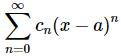where a and cn are numbers. The cn’s are often called the coefficients of the series. The first thing to notice about a power series is that it is a function of x.That is different from any other kind of series that we’ve looked at to this point. In all the prior sections we’ve only allowed numbers in the series and now we are allowing variables to be in the series as well. This will not change how things work however. Everything that we know about series still holds.
In the discussion of power series convergence is still a major question that we’ll be dealing with. The difference is that the convergence of the series will now depend upon the values of x that we put into the series. A power series may converge for some values of x and not for other values of x.
Before we get too far into power series there is some terminology that we need to get out of the way.
First, as we will see in our examples, we will be able to show that there is a number R so that the power series will converge for, |x−a|<R and will diverge for |x−a|>R. This number is called the radius of convergence for the series. Note that the series may or may not converge if |x−a|=R. What happens at these points will not change the radius of convergence.
Secondly, the interval of all x’s, including the endpoints if need be, for which the power series converges is called the interval of convergence of the series.
These two concepts are fairly closely tied together. If we know that the radius of convergence of a power series is R then we have the following.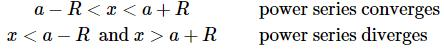The interval of convergence must then contain the interval a−R<x<a+R since we know that the power series will converge for these values. We also know that the interval of convergence can’t contain x’s in the ranges x<a−R and x>a+R since we know the power series diverges for these value of x. Therefore, to completely identify the interval of convergence all that we have to do is determine if the power series will converge for x=a−R or x=a+R. If the power series converges for one or both of these values then we’ll need to include those in the interval of convergence.
Before getting into some examples let’s take a quick look at the convergence of a power series for the case of x=a. In this case the power series becomes,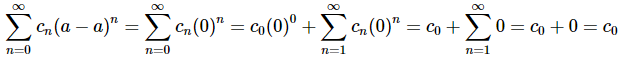and so the power series converges. Note that we had to strip out the first term since it was the only non-zero term in the series.
It is important to note that no matter what else is happening in the power series we are guaranteed to get convergence for x=a. The series may not converge for any other value of x, but it will always converge for x=a.
Let’s work some examples. We’ll put quite a bit of detail into the first example and then not put quite as much detail in the remaining examples.

Example 1: Determine the radius of convergence and interval of convergence for the following power series.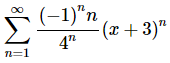Solution. Okay, we know that this power series will converge for x=−3, but that’s it at this point. To determine the remainder of the x’s for which we’ll get convergence we can use any of the tests that we’ve discussed to this point. After application of the test that we choose to work with we will arrive at condition(s) on x that we can use to determine which values of x for which the power series will converge and which values of x for which the power series will diverge. From this we can get the radius of convergence and most of the interval of convergence (with the possible exception of the endpoints).
With all that said, the best tests to use here are almost always the ratio or root test. Most of the power series that we’ll be looking at are set up for one or the other. In this case we’ll use the ratio test.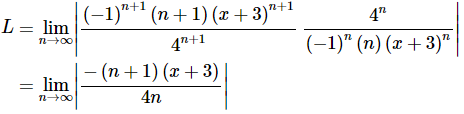Before going any farther with the limit let’s notice that since x is not dependent on the limit it can be factored out of the limit. Notice as well that in doing this we’ll need to keep the absolute value bars on it since we need to make sure everything stays positive and x could well be a value that will make things negative. The limit is then,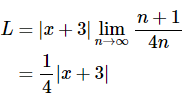So, the ratio test tells us that if L<1 the series will converge, if L>1 the series will diverge, and if L=1 we don’t know what will happen. So, we have,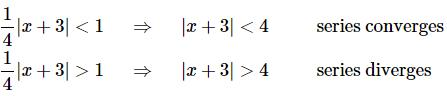We’ll deal with the L=1 case in a bit. Notice that we now have the radius of convergence for this power series. These are exactly the conditions required for the radius of convergence. The radius of convergence for this power series is R=4.
Now, let’s get the interval of convergence. We’ll get most (if not all) of the interval by solving the first inequality from above.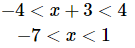So, most of the interval of validity is given by −7<x<1. All we need to do is determine if the power series will converge or diverge at the endpoints of this interval. Note that these values of x will correspond to the value of x that will give L=1.
The way to determine convergence at these points is to simply plug them into the original power series and see if the series converges or diverges using any test necessary.
x=−7:
In this case the series is,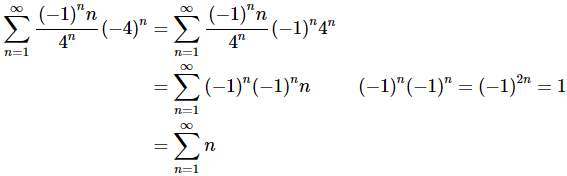This series is divergent by the Divergence Test since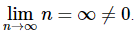x=1 :
In this case the series is,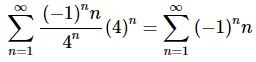This series is also divergent by the Divergence Test since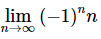doesn’t exist.
So, in this case the power series will not converge for either endpoint. The interval of convergence is then,
−7<x<1
In the previous example the power series didn’t converge for either endpoint of the interval. Sometimes that will happen, but don’t always expect that to happen. The power series could converge at either both of the endpoints or only one of the endpoints.

Example 2: Determine the radius of convergence and interval of convergence for the following power series.
Solution. Let’s jump right into the ratio test.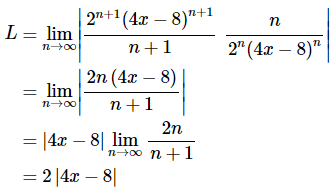So we will get the following convergence/divergence information from this.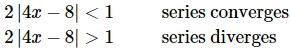We need to be careful here in determining the interval of convergence. The interval of convergence requires |x−a|<R and |x−a|>R. In other words, we need to factor a 4 out of the absolute value bars in order to get the correct radius of convergence. Doing this gives,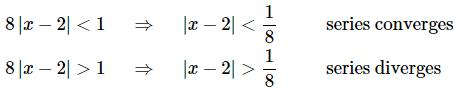So, the radius of convergence for this power series is R=1/8.
Now, let’s find the interval of convergence. Again, we’ll first solve the inequality that gives convergence above.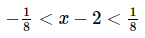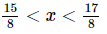Now check the endpoints.
x = 15/8.
The series here is,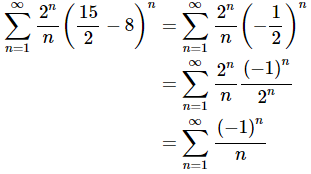This is the alternating harmonic series and we know that it converges.
x = 17/8.
The series here is,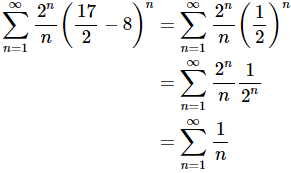This is the harmonic series and we know that it diverges.
So, the power series converges for one of the endpoints, but not the other. This will often happen so don’t get excited about it when it does. The interval of convergence for this power series is then,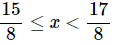We now need to take a look at a couple of special cases with radius and intervals of convergence.

Example 3: Determine the radius of convergence and interval of convergence for the following power series.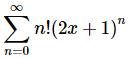Solution. We’ll start this example with the ratio test as we have for the previous ones.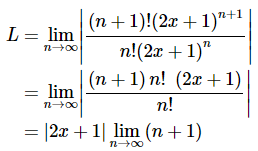At this point we need to be careful. The limit is infinite, but there is that term with the x’s in front of the limit. We’ll have L=∞>1 provided x≠−1/2.
So, this power series will only converge if x=−1/2. If you think about it we actually already knew that however. From our initial discussion we know that every power series will converge for x=a and in this case a=−1/2. Remember that we get a from (x−a)n, and notice the coefficient of the x must be a one!
In this case we say the radius of convergence is R=0 and the interval of convergence is x=−1/2, and yes we really did mean interval of convergence even though it’s only a point.

Example 4: Determine the radius of convergence and interval of convergence for the following power series.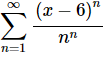Solution. In this example the root test seems more appropriate. So,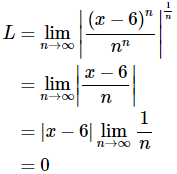So, since L=0<1 regardless of the value of x this power series will converge for every x.
In these cases, we say that the radius of convergence is R=∞ and interval of convergence is −∞<x<∞.
So, let’s summarize the last two examples. If the power series only converges for x=a then the radius of convergence is R=0 and the interval of convergence is x=a. Likewise, if the power series converges for every x the radius of convergence is R=∞ and interval of convergence is −∞<x<∞.
Let’s work one more example.

Example 5: Determine the radius of convergence and interval of convergence for the following power series.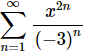Solution. First notice that a=0 in this problem. That’s not really important to the problem, but it’s worth pointing out so people don’t get excited about it.
The important difference in this problem is the exponent on the x. In this case it is 2n rather than the standard n. As we will see some power series will have exponents other than an n and so we still need to be able to deal with these kinds of problems.
This one seems set up for the root test again so let’s use that.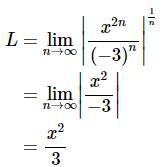So, we will get convergence if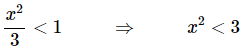The radius of convergence is NOT 3 however. The radius of convergence requires an exponent of 1 on the x. Therefore,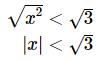Be careful with the absolute value bars! In this case it looks like the radius of convergence is R=√3.Notice that we didn’t bother to put down the inequality for divergence this time. The inequality for divergence is just the interval for convergence that the test gives with the inequality switched and generally isn’t needed. We will usually skip that part.
Now let’s get the interval of convergence. First from the inequality we get,
−√3<x<√3.
Now check the endpoints.
x=−√3:
Here the power series is,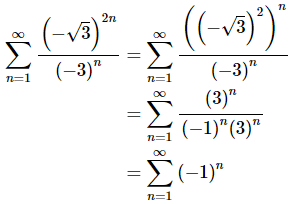This series is divergent by the Divergence Test since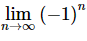doesn’t exist.
x=√3:
Because we’re squaring the x this series will be the same as the previous step.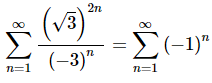which is divergent.
The interval of convergence is then,
−√3<x<√3.

The document Power Series Mathematics Notes | EduRev is a part of the Mathematics Course Algebra for IIT JAM Mathematics.
All you need of Mathematics at this link: MathematicsUse Code STAYHOME200 and get INR 200 additional OFF Use Coupon Code
All Tests, Videos & Notes of Mathematics: Mathematics## Algebra for IIT JAM Mathematics

70 videos|58 docs

Track your progress, build streaks, highlight & save important lessons and more!

,

,

,

,

,

,

,

,

,

,

,

,

,

,

,

,

,

,

,

,

,

;